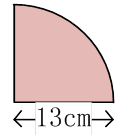### Sample Problem

Find the area of the quarter-circle below.  Use 3.14 for π and round the answer to the nearest hundredth.The area of the quarter-circle is  cm2

#### Solution

The area of the circle is:

3.14 × 13 × 13 = 530.66 cm2

Soof the area of the circle is (Correct to 2 decimal places) :

530.66 ÷ 4 = 132.67 cm2